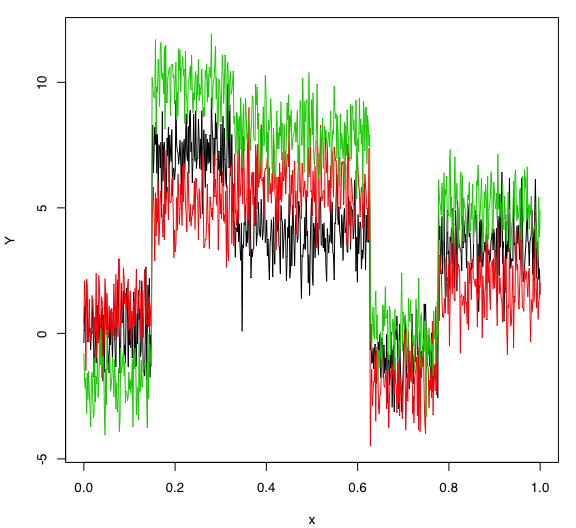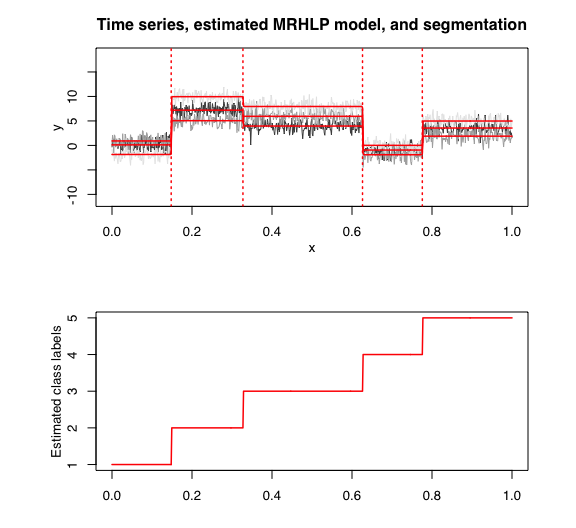# Introduction

In this package, it is possible to select models based on information criteria such as BIC, AIC and ICL.

The selection is done on two parameters which are:

• $$K$$: The number of regimes;
• $$p$$: The order of the polyniomial regression.

# Data

Let’s select a MRHLP model for the following multivariate time series $$Y$$:

data("multivtoydataset")
x <- multivtoydataset$x y <- multivtoydataset[, c("y1", "y2", "y3")] matplot(x, y, type = "l", xlab = "x", ylab = "Y", lty = 1)# Model selection with BIC selectedmrhlp <- selectMRHLP(X = x, Y = y, Kmin = 2, Kmax = 6, pmin = 0, pmax = 3) ## The MRHLP model selected via the "BIC" has K = 5 regimes ## and the order of the polynomial regression is p = 0. ## BIC = -3033.20042397111 ## AIC = -2913.75756459291 The selected model has $$K = 5$$ regimes and the order of the polynomial regression is $$p = 0$$. According to the way $$Y$$ has been generated, these parameters are what we expected. Let’s summarize the selected model: selectedmrhlp$summary()
## ----------------------
## Fitted MRHLP model
## ----------------------
##
## MRHLP model with K = 5 regimes
##
##  log-likelihood nu       AIC     BIC       ICL
##       -2860.758 53 -2913.758 -3033.2 -3032.414
##
## Clustering table:
##   1   2   3   4   5
## 100 120 200 100 150
##
##
## ------------------
## Regime 1 (K = 1):
##
## Regression coefficients:
##
##   Beta(d = 1) Beta(d = 2) Beta(d = 3)
## 1   0.1131005   0.9124035   -1.850509
##
## Covariance matrix:
##
##  1.19064699  0.12700417  0.05496662
##  0.12700417  0.90279499 -0.03272115
##  0.05496662 -0.03272115  0.89086804
## ------------------
## Regime 2 (K = 2):
##
## Regression coefficients:
##
##   Beta(d = 1) Beta(d = 2) Beta(d = 3)
## 1    7.190579    5.049538    9.952361
##
## Covariance matrix:
##
##   1.0723960 -0.18151782 0.12179798
##  -0.1815178  1.05340358 0.01211349
##   0.1217980  0.01211349 0.76527294
## ------------------
## Regime 3 (K = 3):
##
## Regression coefficients:
##
##   Beta(d = 1) Beta(d = 2) Beta(d = 3)
## 1    3.951224    5.941976    7.950232
##
## Covariance matrix:
##
##   1.02880640 -0.05856588 -0.02543545
##  -0.05856588  1.19527262  0.02309638
##  -0.02543545  0.02309638  1.01201958
## ------------------
## Regime 4 (K = 4):
##
## Regression coefficients:
##
##   Beta(d = 1) Beta(d = 2) Beta(d = 3)
## 1  -0.9461282   -1.901665   0.0135667
##
## Covariance matrix:
##
##   0.88092255 -0.02771294 -0.03959332
##  -0.02771294  1.14567525 -0.10726848
##  -0.03959332 -0.10726848  0.89325063
## ------------------
## Regime 5 (K = 5):
##
## Regression coefficients:
##
##   Beta(d = 1) Beta(d = 2) Beta(d = 3)
## 1    3.549319    1.888239    4.981038
##
## Covariance matrix:
##
##  1.1333159 0.25852405 0.03091090
##  0.2585241 1.21453178 0.05663565
##  0.0309109 0.05663565 0.84026581
selectedmrhlp\$plot(what = "estimatedsignal")